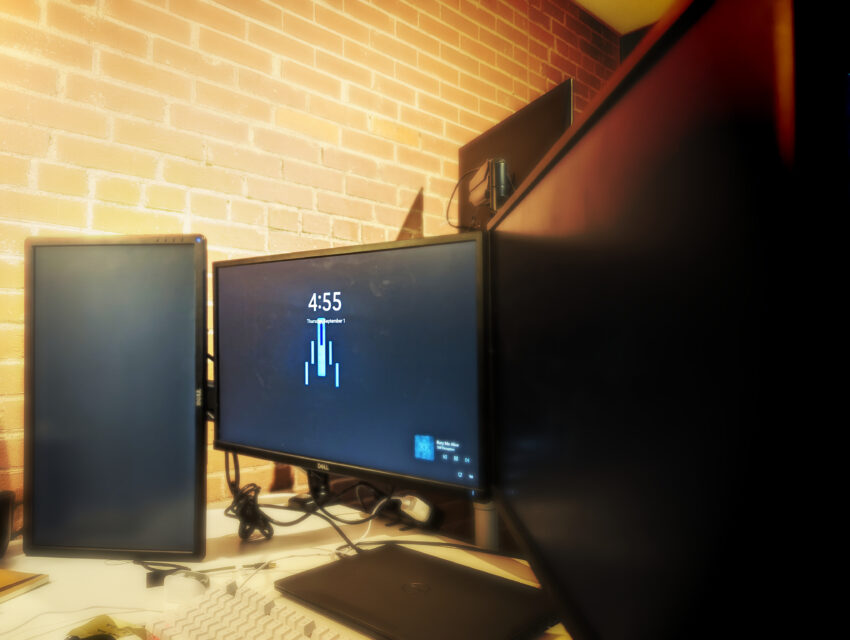# Monitor Discovery Through PowerShellToday, in the field, we will discover a user’s monitors through PowerShell. This is very useful for any kind of RMM tool. I used this script with PDQ and Continuum. You can also run this script with backstage. Let’s take a look at the script for Monitor Discovery.

## The Script

```Get-ciminstance wmimonitorID -namespace root\wmi |
ForEach-Object {
'-2'         = 'Unknown'
'-1'         = 'Unknown'
'0'          = 'VGA'
'1'          = 'S-Video'
'2'          = 'Composite'
'3'          = 'Component'
'4'          = 'DVI'
'5'          = 'HDMI'
'6'          = 'LVDS'
'8'          = 'D-Jpn'
'9'          = 'SDI'
'10'         = 'DisplayPort (external)'
'11'         = 'DisplayPort (internal)'
'12'         = 'Unified Display Interface'
'13'         = 'Unified Display Interface (embedded)'
'14'         = 'SDTV dongle'
'15'         = 'Miracast'
'16'         = 'Internal'
'2147483648' = 'Internal'
}
\$Instance = \$_.InstanceName
\$Sizes = Get-CimInstance -Namespace root\wmi -Class WmiMonitorBasicDisplayParams | where-object { \$_.instanceName -like \$Instance }
\$connections = (Get-CimInstance WmiMonitorConnectionParams -Namespace root/wmi | where-object { \$_.instanceName -like \$Instance }).VideoOutputTechnology
[pscustomobject]@{
Manufacturer   = [System.Text.Encoding]::ASCII.GetString(\$_.ManufacturerName).Trim(0x00)
Name           = [System.Text.Encoding]::ASCII.GetString(\$_.UserFriendlyName).Trim(0x00)
Serial         = [System.Text.Encoding]::ASCII.GetString(\$_.SerialNumberID).Trim(0x00)
Size           = ([System.Math]::Round(([System.Math]::Sqrt([System.Math]::Pow(\$Sizes.MaxHorizontalImageSize, 2) + [System.Math]::Pow(\$_.MaxVerticalImageSize, 2)) / 2.54), 0))
}
}
```

## The Breakdown of Monitor Discovery

### WmimonitorID

The first part of this script is grabbing information from the root namespace. We are looking at the wmi monitor ID information. We are using the get-ciminstance command to grab this information. the wmimonitorid produces encoded data streams. Finally, I pipe the output into a “for each object” loop. This loop is extremely important. By holding everything inside this loop, products like azure PowerShell terminal or Continuum Connectwise see everything after it as part of the initial line.

```Get-ciminstance wmimonitorID -namespace root\wmi | foreach-object {}
```

Aftward, we build out our adapter list inside the loop. I was about to find this list thanks to magnumdb. We set the interface number as the variable name and the type as that variable’s value. This way we can use a simple period call for the type later.

```\$adapterTypes = @{ #https://www.magnumdb.com/search?q=parent:D3DKMDT_VIDEO_OUTPUT_TECHNOLOGY
'-2'         = 'Unknown'
'-1'         = 'Unknown'
'0'          = 'VGA'
'1'          = 'S-Video'
'2'          = 'Composite'
'3'          = 'Component'
'4'          = 'DVI'
'5'          = 'HDMI'
'6'          = 'LVDS'
'8'          = 'D-Jpn'
'9'          = 'SDI'
'10'         = 'DisplayPort (external)'
'11'         = 'DisplayPort (internal)'
'12'         = 'Unified Display Interface'
'13'         = 'Unified Display Interface (embedded)'
'14'         = 'SDTV dongle'
'15'         = 'Miracast'
'16'         = 'Internal'
'2147483648' = 'Internal'
}
```

Next, it’s time to grab additional information. Firstly, we are grabbing the instance name. Later, you will see why.

```\$Instance = \$_.InstanceName
```

Notice we are using the dollar sign followed by an underline. This means grabbing the previous piped item. In our case, the previous item is the wmimonitorid information.

Aftward, we are grabbing the basic sizes of the screen. We are doing this using the wmi object called wmimonitorbasicdisplayparam. Let me untie my tongue after that one. This cim instance produces all of the monitors’ information at once. Thus, we need to filter with a where-object. This command produces the same instance name as the previous command. That’s why we gathered that information beforehand.

```\$Sizes = Get-CimInstance -Namespace root\wmi -Class WmiMonitorBasicDisplayParams | where-object { \$_.instanceName -like \$Instance }
```

Before we continue, I want you to look closely. Notice that we have inside the where-object a \$_. already for the instance name. The \$_ is calling the previous pipe. In this case that is the wmimonitorbasicdisplayparams. This is why we created the \$Instance variable.

Certainly, we will use this same trick with our next piece of information. We will query the wmimonitorconnectionparams using the same method. However, we want only the video output technology variable. This is why we wrap the command inside a parenthesis.

```\$connections = (Get-CimInstance WmiMonitorConnectionParams -Namespace root/wmi | where-object { \$_.instanceName -like \$Instance }).VideoOutputTechnology
```

### Building the Output

Finally, we need to build the output. This is what the end user will see and interact with. This is accomplished by using the ps custom object. Lets talk about each variable as there are special conditions in the code here.

#### Manufacturer

We are using the system text encoding to decode the wmi monitor id data. It will be looking at the manufacturername. After decoding, we want to trim the data.

```[System.Text.Encoding]::ASCII.GetString(\$_.ManufacturerName).Trim(0x00)
```

#### Name

Certainly, like the previous variable, we are going to do the same thing with the name. Using the System text encoding, we get the string and trim down the userFriendlyName information.

```[System.Text.Encoding]::ASCII.GetString(\$_.UserFriendlyName).Trim(0x00)
```

#### Serial

Once again, we will follow the same suit as before. We use the system text encoding to get the string and trim out the results.

```[System.Text.Encoding]::ASCII.GetString(\$_.SerialNumberID).Trim(0x00)
```

#### Size

Afterward, we work with the size. In this case we are going to do some math. The \$Size variable are not encoded like the previous commands. Thus we can do some basic math. We add the max horizontal image size to the max vertical image size. Then we divide that by 2.54. Finally, we round that. This code is a little ugly.

```([System.Math]::Round(([System.Math]::Sqrt([System.Math]::Pow(\$Sizes.MaxHorizontalImageSize, 2) + [System.Math]::Pow(\$_.MaxVerticalImageSize, 2)) / 2.54), 0))
```

#### ConnectionType

Finally, we will use the adapter types from above. It’s as simple as using the \$adaptertypes.”\$connections”. See, I told you that you will see this later.

#### Build the output

```[pscustomobject]@{
Manufacturer   = [System.Text.Encoding]::ASCII.GetString(\$_.ManufacturerName).Trim(0x00)
Name           = [System.Text.Encoding]::ASCII.GetString(\$_.UserFriendlyName).Trim(0x00)
Serial         = [System.Text.Encoding]::ASCII.GetString(\$_.SerialNumberID).Trim(0x00)
Size           = ([System.Math]::Round(([System.Math]::Sqrt([System.Math]::Pow(\$Sizes.MaxHorizontalImageSize, 2) + [System.Math]::Pow(\$_.MaxVerticalImageSize, 2)) / 2.54), 0))
}
```

## Final Thoughts

Monitor Discovery is not out of reach. PowerShell is the tool that brings this to life without costing additional money. I have used this code to grab hundreds of computer monitor information. Then I used a web scraper to grab the prices of the monitors. At the end of the day, I produced a list of computer monitors and their prices. This gave us a total number to give insurance.